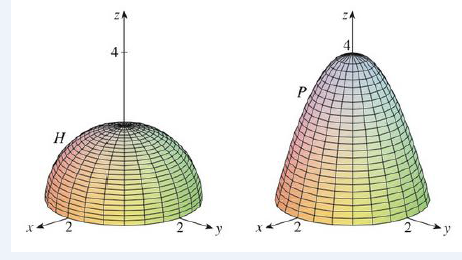Chapter 16.8, Problem 1E

Chapter
Section
Textbook Problem

1. A hemisphere H and a portion P of a paraboloid are shown. Suppose F is a vector field on ℝ3 whose components have continuous partial derivatives. Explain why ∬ H curl   F  ⋅   d S   =   ∬ P curl   F   ⋅   d STo determine

To explain: The reason for satisfying the expression HcurlFdS=PcurlFdS .

Explanation

Given data:

Consider the hemisphere H and a portion P of a paraboloid are shown in the question.

Both hemisphere H and a portion P of a paraboloid are oriented piecewise-smooth surfaces, which are bounded by simple, closed, and smooth curve x2+y2=4 , z=0 .

This means Both surfaces have the same boundary, which is the circle x2+y2=4 .

The hemisphere H and portion P satisfy the hypotheses of Stokes’ theorem since both surfaces are oriented surfaces with the same oriented boundary curve C. Therefore, by Stokes’ theorem, write the following expression,

HcurlFdS=P

Still sussing out bartleby?

Check out a sample textbook solution.

See a sample solution

The Solution to Your Study Problems

Bartleby provides explanations to thousands of textbook problems written by our experts, many with advanced degrees!

Get Started

In Exercises 1-4, simplify the expression. x25x6x29x+18

Calculus: An Applied Approach (MindTap Course List)

In Exercises 6372, evaluate the expression. 72. 2334

Applied Calculus for the Managerial, Life, and Social Sciences: A Brief Approach

limx0sin5x3x=. a) 1 b) 53 c) 35 d) 0

Study Guide for Stewart's Single Variable Calculus: Early Transcendentals, 8th

Sometimes, Always, or Never: fxy = fyx.

Study Guide for Stewart's Multivariable Calculus, 8th